Maths-
General
Easy

Question

# The area of a playground is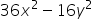. Without removing common factors, factor to possible dimensions of the playground. How are the side-lengths related? What value would you need to subtract from the longer side and add to the shorter side for the playground to be a square?Hint:

## The correct answer is: 6x+4y and 6x-4y are the dimensions of a rectangular playground . 4y needs to be subtracted from the longer side(6x+4y) and adding it to the shorter side(6x-4y) gives the playground to be a square

### Ans :-  6x + 4y and 6x - 4y are the dimensions of a rectangular playground . 4y needs to be subtracted from the longer side(6x + 4y) and adding it to the shorter side(6x - 4y) gives the playground to be a square .Given,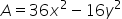Write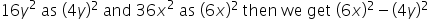As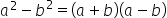Here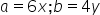We get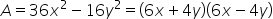As the garden is rectangular , we can write area A =  length × breadthThe possibility is length = 6x + 4y and breadth = 6x - 4y (or) viceversa4y needs to be subtracted from the longer side(6x + 4y) and adding it to the shorter side(6x - 4y) gives the playground to be a square#### With Turito Foundation.#### Get an Expert Advice From Turito.